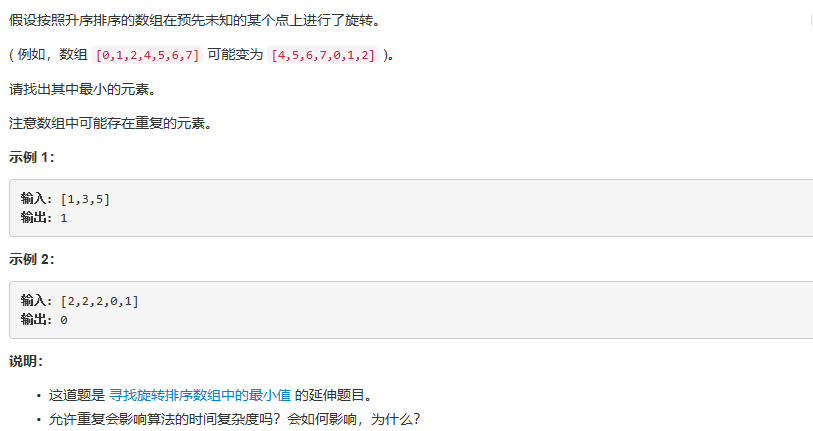• #include int array(int n,int Str[]) ...既然是数字比较，数组用int定义比较好，另外最小值的函数里min最好初始化为Str，因为如果数组里所有数都大于0的话，你的最小值会是0，而不是数组里的数
#include<stdio.h>
int array(int n,int Str[])
{
int j;
//int min=0;
int min=Str;
for(j=0;j<n;j++)
{
if(min>Str[j])
{
min=Str[j];
}
}
return (min);
}

int main()
{
int n;
int i;
int St;
int Min=0;
printf("Please input the number of elements: ");
scanf("%d",&n);
printf("Please input the element: ");
for(i=0;i<n;i++)
{
scanf("%d",&St[i]);
}
Min=array(n,St);
printf("the min number is : %d\n",Min);
return 0;
}
别人给的建议：
既然是数字比较，数组用int定义比较好，另外求最小值的函数里min最好初始化为Str，因为如果数组里所有数都大于0的话，你求到的最小值会是0，而不是数组里的数

展开全文• 把内容过程比较好一些内容片段备份一下，下面内容内容是关于C语言求数组的最大值和最小值的内容，应该能对大伙也有用途。 { { maxValue = a[l] ; minValue = a[l] ; return ; } { if(a[l] &gt;= a...
把内容过程中比较好的一些内容片段备份一下，下面内容内容是关于C语言求数组的最大值和最小值的内容，应该能对大伙也有用途。
{
{
maxValue = a[l] ;
minValue = a[l] ;
return ;
}

{
if(a[l] >= a[r])
{
maxValue = a[l] ;
minValue = a[r] ;
}
else
{
maxValue = a[r] ;
minValue = a[l] ;
}
return ;
}

}

用循环，实现如下：

{
maxValue = a;
minValue = a;
for (int i=1; i<nCount; i++)
{
if (maxValue<a[i])
{
maxValue = a[i];
}
else if (maxValue>a[i])
{
minValue = a[i];
}
}
}

测试代码：

int main()
{

a=2;
a=5;
a=3;
a=4;
a=7;
a=0;

b=8;
b=9;
b=6;

int MaxNum;
int MinNm;

MaxandMin(a, 0, 5, MaxNum, MinNm);

cout << "MinNm=" << MinNm << ",MaxNum=" << MaxNum <<endl;

MaxandMinByLoop(b, 3, MaxNum, MinNm);

cout << "MinNm=" << MinNm << ",MaxNum=" << MaxNum <<endl;

delete[] a;
a=NULL;
delete[] b;
b=NULL;

cout << endl;

return 0;
}



展开全文最大值
• c语言上机考试专用，简单易操作，二维数组的最大最小值及行列号
• 利用C语言可以实现对数组各种操作，如输入数组元素，输出数组元素、求数组元素平均值、输出数组元素最大值、输出数组元素最小值、查找某数值元素是否存在、给数组元素排序等功能。本压缩文件是上述功能对应...
• 但是当Mindex与Rindex和Lindex下标代表元素都相等时，这时使用就不能使用二分查找，需要将这一段区间单独取出来使用顺序遍历方法算出数组中最小值。 代码如下： //顺序遍历最小值 int MinInorder(int* ...
题目描述：解题思路：当数组有重复元素时，只要Mindex与Rindex和Lindex下标代表的元素都不相等时还是可以继续使用二分查找，但是当Mindex与Rindex和Lindex下标代表的元素都相等时，这时使用就不能使用二分查找，需要将这一段区间单独取出来使用顺序遍历的方法算出数组中最小值。

代码如下：

//顺序遍历求出最小值
int MinInorder(int* Nums,int NumsSize){
int ret=0;
for(int i=0;i<NumsSize;i++){
if(Nums[ret]>Nums[i])
ret=i;
}
return Nums[ret];
}
int findMin(int* nums, int numsSize) {
assert(nums||numsSize<=0);
int Lindex=0;
int Rindex=numsSize-1;
int Mindex=Lindex;
//当左边值大于右边值时，且Rindex>Lindex,则继续二分查找
while(nums[Lindex]>=nums[Rindex]&&Rindex>Lindex){
//当Lindex和Rindex相邻时，更新Mindex，并跳出循环
if(Rindex-Lindex==1){
Mindex=Rindex;
break;
}
//更新Mindex
Mindex=Lindex+(Rindex-Lindex)/2;
//若Lindex，Mindex，Rindex三个下标表示的元素的大小都相等时，这时就不能再使用二分查找去
//划分新的区间，要使用顺序遍历的方法求出最小元素
if(nums[Mindex]==nums[Lindex]&&nums[Mindex]==nums[Rindex]){
//返回最小值
return MinInorder(nums+Lindex,Rindex-Lindex);
}
//若中间值大于等于左边值，则证明小区间在Mindex的右边，更新Lindex
else if(nums[Mindex]>=nums[Lindex]){
Lindex=Mindex;
}
//若中间值小于等于右边值，则证明小区间在Mindex的左边, 更新Rindex
else if(nums[Mindex]<=nums[Rindex]){
Rindex=Mindex;
}
}
//返回最小值
return nums[Mindex];
}


剑指offer：面试题8：旋转数组最小值
展开全文• 问题：用分治法来求解一个数组最大最小值 分析：用遍历方法来求解当然很简单，但是题目给我们要求是用分治法，因此我们需要每次利用递归来求数组的一个小部分最大最小值，递归结束条件是数组中有两个元素...
问题：用分治法来求解一个数组的最大最小值

分析：用遍历的方法来求解当然很简单，但是题目给我们的要求是用分治法，因此我们需要每次利用递归来求数组的一个小部分的最大最小值，递归的结束条件是数组中有两个元素或者一个元素。

下面是C语言源代码：（在Linux Ubuntu下gcc 4.0 编译通过 ）

#include <stdio.h>

// find the max and min value between a[m] and a[n]
void max_min(int a[],int m, int n, int* max,int* min)
{
int middle,hmax,hmin,gmax,gmin;

if( m==n )
{
*max = * min = a[m];
}
else if(m == n-1)
{
if( a[m] > a[n])
{
*max = a[m];
*min = a[n];
}
else
{
*max = a[n];
*min = a[m];
}
}
else
{
middle = (m+n)/2;
max_min(a,m,middle,&gmax,&gmin);
max_min(a,middle+1,n,&hmax,&hmin);
if(gmax > hmax)
{
*max = gmax;
}
else
{
*max = hmax;
}
if(gmin < hmin)
{
*min = gmin;
}
else
{
*min = hmin;
}
}
}

int main(int argc,char * argv[])
{
int max,min;
int num_elem = argc-1;
int i;
int * array = (int *)malloc( (argc-1) *sizeof(int) );
for(i=1;i<argc;i++)
{
array[i-1]= atoi(argv[i]);
}

max_min(array,0,num_elem-1,&max,&min);

printf("The max is %d ,the min is %d /n",max,min);
return 0;
}
展开全文ubuntu linux gcc 语言 c
• 求数组中最大值、最小值(C语言)#include<stdio.h>void main(void) { int num,i,imax,imin,imaxp,iminp; for(i=0;i;i++) { scanf("%d",&num[i]); } imaxp=iminp=0; imax=imin=num;
• 一、方法一 #include <stdio.h> #define P 5//数组元素的个数 int main() { int i,max1,max2=0;...//初始化：将数组中的元素值赋给最大值 for(i=0;i<P;i++)//遍历数组中的元素 { //条件判断排序算法
• #include//stdio.h是c标准i/o库，是以函数方式向buffer写入或读取字符，iostream.h是c++标准i/o库，引入了输入/输出流概念，是一个类库，是以类方法从streambuf读取，写入字符。 int max=0; int min=...c C gcc GCC
• *找任意数组中的N个最小值的位置 *array:目标数所在的数组 *arrLen:目标数组的长度 *mins:存放结果的数组 *minLen:想要的最小值个数 * **/ void function(int array[],int arrLen,int mins[],int minLe插入排序
•  思路：如果能用两个辅助数组，那么相对来说简单一点，可定义数组Min和数组Max，其中Min[i]表示自a[i]之后的最小值（包括a[i]），Max[i]表示自a[i]之前元素的最大值。有了这两个辅助数组后，对于a[i]，如果它大于...
• 这道题既然是要求方差，那么考虑到方差特性，三个相邻数差值越小那么方差值也就越小，所以这道题解决思路应该是先对数组排序，这样就保证了所选得三个连续得数字拿到得都是差值最小得数，然后每三个一组求得最...编程题
• 求C语言题目，要用到指针谢谢！...用一个函数数组中数据最大值和最小值 函数流程 【获取数据】主函数送来数组名、元素个数 【处理数据】出最大值和最小值的下标 【产生结果】最大值和最小值的下标
• 找出在一行中的最大值，确定最大值所在的列，再出在该列中的最小值所在的行数，比较行是否相等，判断是否为鞍点。 代码： #include <stdio.h> #include <stdlib.h> int main() { int n,m,i,j; ...算法
• ##题目一 数组中偶数和 1.实验代码 #include&lt;stdio.h&gt; int main() { int i,sum=0; int a; for(i=0;i&lt;10,i++) { scanf("%d",&amp;a[i]); if(a[i]%2==0){ ...数组中偶数的和 输出小于均值的数
• #define COUNT 10int main(int argc, const char * argv[]){ //1、随机产生20个[10 , 50]的正整数存到数组中，并求数组中的所有元素最大值、最小值、平均值以及各元素之和。第二大值。// int max = 0, min = 0...
• #include<... printf("输入数组元素个数：\n"); scanf("%d",&n); printf("输入%d个整数：\n",n); if(n<1||n>100) return 0;//程序读到return后就不再执行 for(i=0;i<n;i++...
• 根据用户输入10人成绩并将其保存到数组中最高成绩，最低成绩和平均成绩 int scoure;//存储10个数据数组 int i; int sum;//总成绩 int max,min,avg;//最大值，最小值，平均成绩 for(i=0;i<10;i++...
• 二维数组中的数在主函数中赋予。 //重难点：出的是每一列的最小值，这里要注意，学会简化代码，省去一些多余定义的变量。 1 #include <stdio.h> 2 #define M 3 3 #define N 4 4 void fun ( ...
• ## C语言数组之排序

千次阅读 2019-03-25 19:56:34
基本过程是先将第一个数分别于后面数一个一个进行比较，若后面数小，则交换后面这个数和第一个数位置，否则不交换，一轮比较结束后就出了一个最小值的数放在了第一位。然后进入第二轮比较，即在其余再...# c语言求数组中的最小值c语言 订阅